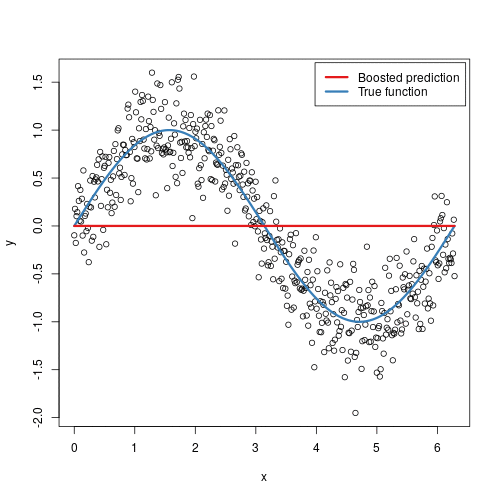It is a machine learning method for regression and classification problems. The gradient boosting model is a linear …

Oct. 13, 2022 1 minuteIt is a machine learning method for regression and classification problems. The gradient boosting model is a linear combination of weak models periodically built to create a robust final model.

Decision trees or methods based on bagging, such as a random forest, are better models for soft models. The accuracy of the gradient boosting model is partially affected by the characteristics of the input data.

The accuracy of a predictive model can be strengthened in two ways:

a) Using feature engineering.

b) By directly applying the boosting algorithms.

There are many boosting algorithms, including Gradient Boosting, XGBoost, AdaBoost, Gentle Boost, etc.; each has its basic mathematics. Also, they have minor changes to each other that will be determined during implementation.## How is XGBoost different from gradient boosting?

XGBoost has a very high predictive power, making it the best option for accuracy in various events because it has a linear model and a tree learning algorithm.

This algorithm is approximately ten times faster than existing gradient boosting algorithms. This algorithm includes various objective functions, regression, classification, and ranking.

One of the most exciting things about XGBoost is that it is also known as the regularized boosting technique. This algorithm helps reduce large models and has good support in various languages such as Scala, Java, R, Python, Julia, and C++.The models in Gradient Boosting Machine are built sequentially, and each of these subsequent models tries to reduce the error of the previous model. But the question is, how does each model reduce the error of the previous model?

It is done by building the new model over errors or residuals of the previous predictions. This is done to determine any patterns in the error that the previous model misses.

The Boosting Grading algorithm can easily overfit a training data set, which different constraints or regularization methods can mitigate.

## How does gradient boosting work for classification?

Gradient boosting consists of three main components:

1. Loss Function: A model's performance is determined by the loss function based on how well it predicts.
2. Weak Learner: Weak learners (such as decision trees) poorly classify data.
3. Additive Model: Incorporates weak learners sequentially and iteratively. The loss function value should decrease with each iteration, bringing us closer to the final model.

## What gradient boosted decision trees?

Gradient-boosted decision trees are machine learning techniques for optimizing the predictive value of a model through successive steps.

A decision tree is iterated with the coefficients, weights, or biases applied to each input variable to predict the target value to minimize the loss function (the difference between predicted and actual target values).

Gradients refer to incremental improvements in predictive accuracy made at each process step. Boosting involves increasing predictive accuracy to reach the optimal level.## Conclusion

Gradient Boosting has repeatedly proven one of the most powerful techniques for building predictive models in classification and regression. Many of the real-life machine learning challenges have been solved by Gradient Boosting.